Next: References Up: Luminosity Spectrum Previous: Measurement of the Luminosity

## Beam Parameter Fitting

Theoretically, the luminosity spectrum is determined by a set of beam parameters: the horizontal, vertical and longitudinal beam sizes (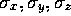) and the number of particles in a bunch(N). In the empirical function, two independent-valuables appeared as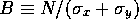and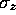. They can be calculated from the measured spectrum, since the measured one is the luminosity spectrum convoluted by the cross section of the Bhabha scattering process, which is called the weighted luminosity spectrum. In this analysis, one of them is obtained by assuming a fixed value of the other, and vice versa, i.e. one parameter fitting in a likelihood method. The beam energy was set to be 250 GeV and the relevant parameters are listed in Table 14.1, which are called ``nominal" beam parameters.

For this study, two kinds of Bhabha events were generated by the method described in the previous section. They are called an `experimental-data sample' (e-sample) and a `likelihood-function sample' (l-sample). The Monte Carlo statistics of e-samples corresponds to 10fb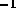and 1fbfor the angular regions of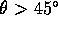and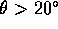, respectively. The polar angles of electrons and positrons in the Bhabha events were smeared with a Gaussian distribution of 1mrad resolution; then, the weighted luminosity-spectrum was calculated as a function of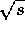from their acollinearity angles. A number of e-samples were generated with several sets of B and. To determine a likelihood function, a l-sample was generated with 10 times more statistics than the e-samples with the nominal beam parameters. The weighted luminosity spectrum was obtained in the same way as the e-sample. Dividing the spectrum into 50 bins for 450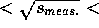500GeV, the normalized likelihood function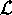was defined by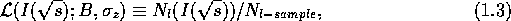where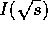is the bin-number corresponding to,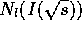is the number of events in the'th bin, and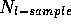is the total event number of the l-sample. For the e-sample, the log-likelihood was calculated by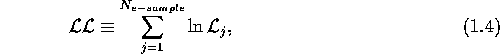where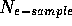is the total event number of the e-sample and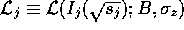is the likelihood of the j'th event in the e-sample.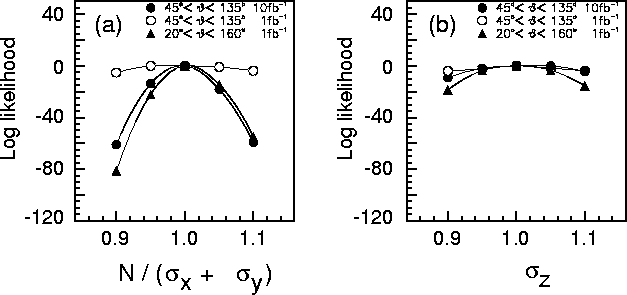Figure 14.23: Log-likelihood distributions of the e-samples as a function of (a)and (b), where the parameter was varied for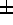10% relative to the nominal value, while the other was fixed to the nominal value and vice versa.

Figures 14.23(a) and (b) show the log-likelihood distributions as a function of B and, respectively. From these figures, it can be seen that the beam parameter of B orcan be determined with an accuracy of several % by large () and small() angle Bhabha events for an integrated luminosity of 10fband 1fb, respectively.

As described in the previous section, since the energy scan should be made for the integrated luminosity of a few fbeach at a number of CM energies around toponium resonance states, a 1 mrad angular resolution must be necessary in the small angular region (). In these cases, the integrated luminosity of the peak in the luminosity spectrum must be precisely determined, although the peak may be smeared by the beam energy spread. It is seen that the present study has demonstrated the feasibility toward this end.Next: References Up: Luminosity Spectrum Previous: Measurement of the Luminosity

Toshiaki Tauchi
Thu, May 29, 1997 04:47:48 PM